Impact Factor 2.680 | CiteScore 3.57
More on impact ›

# Frontiers in Neuroinformatics## Original Research ARTICLE

Front. Neuroinform., 22 June 2017 | https://doi.org/10.3389/fninf.2017.00039

# Bioinspired Architecture Selection for Multitask Learning

• 1Department of Computer Science, Universidad Católica de Murcia, Murcia, Spain
• 2Department of Information and Communications Technologies, Universidad Politécnica de Cartagena, Cartagena, Spain

## 1. Introduction

The Hebbian learning in neural networks consists in establishing new synapses according to new lived experiences. Thus, this learning is directly related to the so-called structural plasticity which is the brain's ability to alter their physical structure in response to the learning of new information, skills, or habits. This means that when a human being modifies the knowledge about a particular field with new information (both from the same field and from other related fields), new neural connections are established and others are inhibited. This is how human beings can improve knowledge on a specific topic: by incorporating new experiences or related knowledge.

In Bueno-Crespo et al. (2015), a method to select related tasks with the main one is presented. Now, this method is used as a part of a new procedure to completely design MTL architectures. A particular pruning connections procedure leads to a positive transfer of information from the secondary tasks to the main task because only the most relevant connections are preserved. In this sense, the proposed method performs a complete design of the MTL networks. To achieve this, the method takes advantage of the benefits of Extreme Learning Machine algorithm (ELM) (Huang et al., 2006), specifically the Optimally Pruned ELM (OP-ELM) (Miche et al., 2010), and the Architecture Selection based on ELM (ASELM) procedures (Bueno-Crespo et al., 2013).

The rest of the paper is organized as follows: Section 2. describes the ASELM algorithm to design Multilayer Perceptrons (MLP). A summarized description of MTL is presented in Section 3. The proposed method is described in Section 4. Section 5 shows the results and finally, conclusions and prospective works close the paper.

## 2. Architecture Selection Using Extreme Learning Machine

The Extreme Learning Machine (ELM) is based on the concept that if the MLP input weights are fixed to random values, the MLP can be considered as a linear system and the output weights can be easily obtained by using the pseudo-inverse of the hidden neurons outputs matrix H for a given training set. Although related ideas were previously analyzed in other works (Pao et al., 1994; Igelnik and Pao, 1997), Huang was the author who formalized it (Huang and Chen, 2007; Huang et al., 2011). He demonstrated that the ELM is an universal approximator for a wide range of random computational nodes, and all the hidden node parameters can randomly be generated according to any continuous probability distribution without any prior knowledge. Thus, given a set of N input vectors, a MLP can approximate N cases with zero error, $\sum _{i=1}^{N}||{\text{y}}_{i}-{\text{t}}_{i}||=0$, being ${\text{y}}_{i}\in {ℝ}^{m}$ the output network for the input vector ${\mathbf{\text{x}}}_{i}\in {ℝ}^{n}$ with target vector ${\text{t}}_{i}\in {ℝ}^{m}$. Thus, there exist ${\beta }_{j}\in {ℝ}^{m}$, ${\mathbf{\text{w}}}_{j}\in {ℝ}^{n}$ and bj ∈ ℝ such that,

where ${\beta }_{j}={\left[{\beta }_{j1},{\beta }_{j2},\dots ,{\beta }_{jm}\right]}^{T}$ is the weight vector connecting the jth hidden node with the output nodes, ${\mathbf{\text{w}}}_{j}={\left[{w}_{j1},{w}_{j2},\dots ,{w}_{jn}\right]}^{T}$ is the weight vector connecting the jth hidden node with the input nodes, and bj is the bias of the jth hidden node.

For a network with M hidden nodes, the previous N equations can be expressed by

where

where H ∈ ℝN×M is the hidden layer output matrix of the MLP, B ∈ ℝM×m is the output weight matrix, and T ∈ ℝN×m is the target matrix of the N training cases. Thus, as wj and bj with j = 1, …, N, are randomly selected, the MLP training is given by the solution of the least square problem of Equation (2), i.e., the optimal output weight layer is $\stackrel{^}{\mathbf{\text{B}}}={\mathbf{\text{H}}}^{‡}\mathbf{\text{T}}$, where H is the Moore-Penrose pseudo-inverse (Serre, 2002).

ELM for training MLPs can be therefore summarized as shown in Algorithm 1.

ALGORITHM 1

ELM provides a fast and efficient MLP training (Huang et al., 2006), but it needs to fix the number of hidden neurons to obtain a good generalization capability. In order to avoid the exhaustive search for the optimal value of M, several pruned methods have been proposed (Mateo and Lendasse, 2008; Miche et al., 2008a,b; Rong et al., 2008; Miche and Lendasse, 2009; Miche et al., 2010). Among them, the most commonly used is the ELM Optimally Pruned (OP-ELM) (Miche et al., 2010). The OP-ELM sets a very high initial number of hidden neurons (MN) and, by using Least Angle Regression algorithm (LARS) (Similä and Tikka, 2005), sorts the neurons according to their importance to solve the problem (Equation 2). The pruning of neurons is done by utilizing Leave-One-Out Cross-Validation (LOO-CV) and choosing the combination of neurons (which have been previously sorted by the LARS algorithm) that provides lower LOO error. The LOO-CV error is efficiently computed using the Allen's formula (Miche et al., 2010). For more detail, a summary of the OP-ELM algorithm is shown in Algorithm 2 (García-Laencina et al., 2011).

ALGORITHM 2

Recently, a new method to design MLP architectures has been presented in Bueno-Crespo et al. (2013). It is called ASELM (“Architecture Selection Using Extreme Learning Machine”) and is based on the OP-ELM. Thus, once the initial MLP architecture is defined, the OP-ELM optimally discards those hidden neurons whose combination of input variables is not relevant to the target task. Because of the binary value of the input weights, the selection of hidden nodes implies also the selection of those relevant connections between the input and hidden layers. Thus, only input connections corresponding to selected hidden neurons and with input weights values equal to 1 will be part of the final architecture. A summary of the ASELM algorithm is shown below (Algorithm 3).

ALGORITHM 3

The MTL architecture for a neural network is similar to the classical scheme STL (Single Task Learning). They differ in that MTL scheme has an output for each task to be learned, whereas STL scheme has a separate network for each one (Figure 1). Thus, when we speak about MTL, we are referring to a type of learning where a main task and other tasks (considered as secondary tasks) are learned all at once in order to help learning of the main one.

FIGURE 1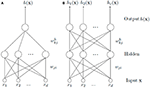Figure 1. Different learning schemes. In (A), a STL architecture is shown. It is used to solve a single task alone. In (B), a set of tasks are learned simultaneously by means of a MTL architecture. In this case, there is a common part (from the input to the hidden layer) and a specific part (from the hidden layer to the output) for each task.

In a MTL scheme, there is a common part shared by all tasks and a specific one for each task. The common part is formed by the weights connections from the input features to the hidden layer, allowing common internal representation for all tasks (Caruana, 1993). Thanks to this internal representation, learning can be transferred from one task to another (Caruana, 1998). The specific part, formed by the weights that connect the hidden layer to the output layer, specifically allows modeling each task from the common representation. The main problem with this type of learning is to find tasks related to the main one. Even in case of finding them, it may be difficult to know the kind of relationship they have, because it can be a positive or negative influence to learn the main task.

## 4. Proposed Method

The method proposed in this paper is called MTLASELM since it is based on the ASELM to design MTL architectures. To do this, it is necessary to introduce a couple of modifications to the original method so as to adapt it to MTL. Firstly, the targets of secondary tasks will be used as new input features (removing them from the outputs of the classic MTL scheme) so that a similar architecture to that shown in Figure 1A is obtained. There is only a single output corresponding to the main task and an input vector composed now by the original input features and the targets of secondary tasks. This network is designed and trained using ASELM which, as it was commented before, realizes a selection of hidden nodes that implies also the selection of those relevant connections between the input and hidden layer. The selection of relevant secondary tasks is now performed since they are part of the input vector.

In a second stage, a MTL architecture is created. The secondary tasks selected in the previous stage as the most relevant to learn the main task are included as output components in the MTL neural network. A scheme of the proposed method can be seen in Figure 2.

FIGURE 2

This idea of exchanging outputs for inputs is not new. Caruana proposed that some inputs may work better as outputs, i.e., as new secondary tasks (Caruana, 1998). This idea is very interesting in machine learning and it has been used, for example, for developing efficient procedures to classify patterns with missing data (García-Laencina et al., 2010, 2013).

MTLASELM method allows pruning to take place both at the hidden layer and the output layer, at the same time that provides a unique solution. This uniqueness comes from the binary initialization of the hidden weights, which eliminates the random component thereof.

To further clarify the MTLASELM method, the following section includes an example of how the method is applied step by step to solve a particular problem (Logic Domain problem).

## 5. Experiments

In order to show the goodness of the MTLASELM method for designing an MTL architecture, results of classification test obtained with the single-task learning (STL), classic multitask learning (MTL), and MTLASELM have been compared. While the MTLASELM architecture is directly obtained by the proposed method, the best architecture for STL and MTL has been selected by cross-validation. For experiments, the three architectures are trained using the stochastic back-propagation with a cross-validation with 10-fold × 30 initializations. “Logic Domain,” “Monk's Problems,” “Telugu,” “Iris Data,” and “User Knowledge Modeling” datasets, will be used to show the performance of the method. These data sets are available at the UCI ML Repository (Asuncion and Newman, 2007), excepting “Logic Domain” problem (McCracken, 2003). Specific details about results for each dataset are described below.

“Logic Domain” dataset is used to see how MTL architecture is created by the MTLASELM method. This dataset is a toy problem specially designed for multitask learning. In this problem, targets are represented by the combination of four real variables (from seven inputs: x1,…,x7), considering the first task as the main task, and the others as secondary ones.

Table 1 shows the logical expression for each task. Note, that the main task (Tp) is only determined by the first four features of the problem. The secondary tasks share one or more variables with the main one. Nevertheless, only the second secondary task (TSec2) shares a common logic subexpression (x3 > 0.5 ∨ x4 > 0.5) with the main task.

TABLE 1

Initially, the neural network architecture is composed by M = 1023 (2n − 1, with n = 10; seven input features + three extra features corresponding to three secondary tasks) hidden units (see Figure 3). This suppose a large enough hidden layer number according to the ELM theory. Once this model is trained with ASELM method, the result is quite significant. The ASELM selects only two hidden neurons as the most relevant to learn the main task (Bueno-Crespo et al., 2013). By relevance order, these hidden weights are w194 = [0 0 1 1 0 0 0 0 1 0] and w768 = [1 1 0 0 0 0 0 0 0 0] corresponding to hidden neuron number 194 and 768, respectively. For simplicity, we will be referred to them as first neuron or w1 and second neuron or w2. From w1, it can be observed that the first selected hidden neuron is only connected to input features x3 and x4, as well as the second secondary task (TSec2). From w2, it follows that only x1 and x2 contribute to learning through their connection to the second hidden neuron (see Figure 3).

FIGURE 3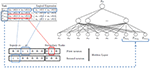Figure 3. Logic Domain problem. Scheme to learn the main task using secondary tasks as inputs. 1023 neurons in the hidden layer have been generated. After ASELM is applied only two hidden neurons are selected whose weight vectors are shown in black boxes. The first neuron has three connections corresponding to the input features x3 and x4 and the second secondary task (TSec2). The second neuron is represented only by the input features x1 and x2.

This means that only TSec2 is influencing in the learning of the TP through the first neuron that learns the input features x3 and x4, which is an expected result according to the previous comment indicating the relationship between Tp and Tsec2 (see Table 1). The second selected hidden neuron is only composed by the input features x1 and x2, without any input connection from the secondary tasks. Figure 4 shows the final architecture given by ASELM method.

FIGURE 4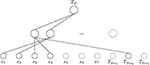Figure 4. Logic Domain problem. Intermediate scheme where connections are pruned after ASELM. It can be observed how the input features x5, x6, and x7, all hidden nodes least two, and secondary tasks TSec1 and TSec3 are removed because they are irrelevant for the learning of the TP.

Next, a MTL architecture is created considering as outputs those corresponding to the main task and secondary ones selected in the previous stage. The latter are incorporated into the output layer preserving the connections established by the ASELM. In our case, only TSec2 has been selected. Figure 5 shows the final MTLASELM architecture. MTLASELM has removed the input features x5, x6, and x7, and has selected only 2 neurons in the hidden layer from the 1023 neurons initially considered.

FIGURE 5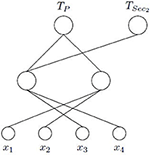Figure 5. Logic Domain problem. Final architecture obtained with MTLASELM. TP shares a hidden layer neuron with TSec2, which learns the common part of both tasks.

Figure 6 shows the MTLASELM schemes for other studied datasets. “Monk's Problems” dataset is a collection of three toy problems that present the same domain (six input features). In this problem, the targets associated to each task are described by the logical relations. Thus, Monk 1 (TP) is described by (x1 = x2) ∨ (x5 = 1); in Monk 2 (TSec1) exactly two identities from x1 = 1, x2 = 1, x3 = 1, x4 = 1, x5 = 1, x6 = 1 must be satisfied; and in Monk 3 (TSec2), (x5 = 3 and x4 = 1) or (x5 ≠ 4 and x2 ≠ 3) have to be fulfilled. MTLASELM selects 14 neurons in the hidden layer from a total of 255. Figure 6A presents the first five neurons and the last one for the selected architecture. For example, if we observe the first neuron, it connects the input feature x5 with the outputs of TP and TSec1, but not with TSec2. It can be observed that target associated to TP and TSec1 match the value of x5, what does not happen for TSec2.

FIGURE 6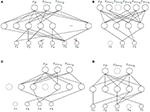Figure 6. Final MTLASELM architectures for “Monk's Problems” (A), “Telugu” (B), “Iris Data” (C), and “User Knowledge Modeling” (D).

“Telugu” language dataset represents one of six languages designated a classical language of India. This datasets consists of three input features that represent language formants. For “Telugu,” MTLASELM selects 4 neurons in the hidden layer from a total of 255 initial neurons. Figure 6B shows the final architecture obtained. As can be seen, this architecture uses only two of the three input features, what is quite interesting because in dialects with fewer than six vowels, two formants are only required to classify (Pal and Majumder, 1977).

“Iris Data” (Figure 6C) represent a dataset of three types of flowers represented by four input features. For this dataset, 5 neurons are selected in the hidden layer from a total of 63 neurons. It can be observed that the input feature x1 has been removed. The results show that the proposed method has a much more simplified architecture than classical multitask learning, although the classification test is similar due to the simplicity of the problem (see Table 2).

TABLE 2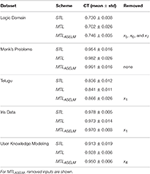Table 2. Classification Test “CT” (mean ± standard deviation) with different schemes on several datasets.

“User Knowledge Modeling” (Figure 6D) is the real dataset about the students' knowledge status about the subject of Electrical DC Machines. The target is represented by four levels (very low, low, middle, and high). To give a multitasking approach a pairwise combination has been made (TP = (very low ∨ low), TSec1 = (low ∨ middle), and TSec2 = (middle ∨ high)). It can be observed that TSec1 is removed. It is because TSec2 is more important to TP, since TSec2 represents its opposite. Finally, 5 neurons are selected in the hidden layer from a total of 127 initial neurons.

Table 2 shows the classification accuracy results for all the data sets. Because the Logic Domain is an easy problem to solve for an MLP in an STL scheme, the number of samples has been reduced to 50 so that multitask learning can be appreciated. With all training samples, the result between STL and MTLASELM is practically invaluable. Taking into account this reduction of samples for the Logic Domain problem, MTLASELM presents better classification accuracy than STL and MTL. However, STL is better than the classic MTL, since MTL presents a completely interconnected scheme that is positively influenced by the related task (TSec2) and negatively by unrelated tasks (TSec1 y TSec3). This is not a general rule but it is an empirical result that shows that there are tasks that help the main task and others that are harmful.

For the rest of the data sets, the MTLASELM always provides the best results on average with a low standard deviation. This robustness in the solution is due to the particular initialization of the hidden weights that MTLASELM realizes.

To validate this assertion, a non-parametric statistical test has been performed. Specifically, the Wilcoxon Signed Ranks Test is used (Kruskal, 1957). A peer review has been performed. Comparing MTLASELM to STL, the p-value obtained is 0.078, which indicates that there are significant differences to 92%, being the best MTLASELM. Likewise, when applying the test with MTLASELM against classic MTL, the p = 0.080 indicates that there are significant differences to 92%, being MTLASELM better than MTL. However, there are no significant differences between STL and MTL, since the p = 0.683.

## 6. Discussion and Future Work

Authors are working on extending the method to other learning models, such as Radial Basis Functions (RBF). Applying MTLASELM to regression problems is another research field since the ASELM is optimized for classification according to Huang et al. (2010). This limitation of the present method is due to the nature of ELM method, which is based on the pseudoinverse calculation. In this regard, we are working to use the sequential calculation pseudoinverse of Moore-Penrose (Van Heeswijk et al., 2011; Tapson and Van Schaik, 2013). Another line of research is to extend this method in the field of Deep Learning since new works on MultiTask Learning have lately appeared, most of them within the scope of Deep Learning (Liu et al., 2015; Thanda and Venkatesan, 2017).

## Author Contributions

AB: Proposed method, software programming, experiments, reviews, results, and conclusions. RMML: Introduction, software programming, contributions of ideas and reviews. RME: Method description, contributions of ideas and reviews. JS: Work direction, architecture selection, contributions of ideas, reviews, conclusions, and future work.

## Conflict of Interest Statement

The authors declare that the research was conducted in the absence of any commercial or financial relationships that could be construed as a potential conflict of interest.

The handling Editor declared a shared affiliation, though no other collaboration, with several of the authors RMML and JS, and the handling Editor states that the process met the standards of a fair and objective review.

## Acknowledgments

This work has been supported by Spanish MINECO under grant TIN2016-78799-P (AEI/FEDER, UE). This paper is an extension of the one presented at the IWINAC 2015 conference (6th INTERNATIONAL WORK-CONFERENCE on the INTERPLAY between NATURAL and ARTIFICIAL COMPUTATION). Dedicated to the memory of Ph.D. Pedro José García-Laencina.

## References

Asuncion, A., and Newman, D.-J. (2007). UCI Machine Learning Repository. Irvine, CA: Department of Information and Computer Science, University of California. Available online at: http://archive.ics.uci.edu/ml/

Baxter, J. (1993). The evolution of learning algorithms for artificial neural networks. Complex Syst.

Bueno-Crespo, A., García-Laencina, P. J., and Sancho-Gómez, J.-L. (2013). Neural architecture design based on extreme learning machine. Neural Netw. 48, 19–24. doi: 10.1016/j.neunet.2013.06.010

Bueno-Crespo, A., Menchón-Lara, R.-M., and Sancho-Gómez, J.-L. (2015). “Related tasks selection to multitask learning schemes,” in International Work-Conference on the Interplay between Natural and Artificial Computation (Elche: Springer), 213–221. doi: 10.1007/978-3-319-18833-1_23

Caruana, R. (1995). Learning many related tasks at the same time with backpropagation. Adv. Neural Inform. Process. Syst. 7, 657–664.

Caruana, R. (1998). “Multitask learning,” in Learning to Learn (Springer), 95–133.

Caruana, R.-A. (1993). “Multitask connectionist learning,” in Proceedings of the 1993 Connectionist Models Summer School (Boulder).

García-Laencina, P.-J., Bueno-Crespo, A., and Sancho-Gómez, J.-L. (2011). “Design and training of neural architectures using extreme learning machine,” in Neurocomputing: Learning, Architectures and Modeling, ed E. T. Mueller (Nova Science Publishers), 119–145.

García-Laencina, P.-J., Sancho-Gómez, J.-L., and Figueiras-Vidal, A.-R. (2010). Pattern classification with missing data: a review. Neural Comput. Appl. 19, 263–282. doi: 10.1007/s00521-009-0295-6

García-Laencina, P.-J., Sancho-Gómez, J.-L., and Figueiras-Vidal, A.-R. (2013). Classifying patterns with missing values using multi-task learning perceptrons. Expert Syst. Appl. 40, 1333–1341. doi: 10.1016/j.eswa.2012.08.057

Huang, G.-B., and Chen, L. (2007). Convex incremental extreme learning machine. Neurocomputing 70, 3056–3062. doi: 10.1016/j.neucom.2007.02.009

Huang, G.-B., Ding, X., and Zhou, H. (2010). Optimization method based extreme learning machine for classification. Neurocomputing 74, 155–163. doi: 10.1016/j.neucom.2010.02.019

Huang, G.-B., Wang, D., and Lan, Y. (2011). Extreme learning machines: a survey. Int. J. Mach. Learn. Cybernet. 2, 107–122. doi: 10.1007/s13042-011-0019-y

Huang, G.-B., Zhu, Q.-Y., and Siew, C.-K. (2006). Extreme learning machine: theory and applications. Neurocomputing 70, 489–501. doi: 10.1016/j.neucom.2005.12.126

Igelnik, B., and Pao, Y.-H. (1997). Stochastic choice of basis functions in adaptive function approximation and the functional-link net. IEEE Trans. Neural Netw. 8, 452–454.

Kruskal, W.-H. (1957). Historical notes on the wilcoxon unpaired two-sample test. J. Am. Stat. Assoc. 52, 356–360. doi: 10.1080/01621459.1957.10501395

Liu, X., Gao, J., He, X., Deng, L., Duh, K., and Wang, Y.-Y. (2015). “Representation learning using multi-task deep neural networks for semantic classification and information retrieval,” in HLT-NAACL (Denver), 912–921. doi: 10.3115/v1/n15-1092

Mateo, F., and Lendasse, A. (2008). “A variable selection approach based on the delta test for extreme learning machine models,” in Proceedings of the European Symposium on Time Series Prediction (Porvoo), 57–66.

McCracken, P.-J. (2003). Selective Representational Transfer Using Stochastic Noise. Honurs thesis, Jodrey School of Computer Science, Acadia University.

Miche, Y., Bas, P., Jutten, C., Simula, O., and Lendasse, A. (2008a). “A methodology for building regression models using extreme learning machine: OP-ELM,” in Proceedings of European Symposium on Artificial Neural Networks (ESANN) (Bruges), 247–252.

Miche, Y., and Lendasse, A. (2009). “A faster model selection criterion for OP-ELM and OP-KNN: Hannan-quinn criterion,” in European Symposium on Artificial Neural Networks (ESANN), Vol. 9 (Bruges), 177–182.

Miche, Y., Sorjamaa, A., Bas, P., Simula, O., Jutten, C., and Lendasse, A. (2010). OP-ELM: optimally pruned extreme learning machine. IEEE Trans. Neural Netw. 21, 158–162. doi: 10.1109/TNN.2009.2036259

Miche, Y., Sorjamaa, A., and Lendasse, A. (2008b). “OP-ELM: theory, experiments and a toolbox,” in International Conference on Artificial Neural Networks (Prague: Springer), 145–154. doi: 10.1007/978-3-540-87536-9_16

Pal, S.-K., and Majumder, D.-D. (1977). Fuzzy sets and decision making approaches in vowel and speaker recognition. IEEE Trans. Syst. Man Cybernet. 7, 625–629. doi: 10.1109/TSMC.1977.4309789

Pao, Y.-H., Park, G.-H., and Sobajic, D.-J. (1994). Learning and generalization characteristics of the random vector functional-link net. Neurocomputing 6, 163–180. doi: 10.1016/0925-2312(94)90053-1

Rong, H.-J., Ong, Y.-S., Tan, A.-H., and Zhu, Z. (2008). A fast pruned-extreme learning machine for classification problem. Neurocomputing 72, 359–366. doi: 10.1016/j.neucom.2008.01.005

Serre, D. (2002). Matrices: Theory and Applications. Graduate Texts in Mathematics. New York, NY: Springer.

Silver, D., and Mercer, R. (2001). “Selective functional transfer: Inductive bias from related tasks,” in IASTED International Conference on Artificial Intelligence and Soft Computing (ASC2001) (Cancum), 182–189.

Similä, T., and Tikka, J. (2005). “Multiresponse sparse regression with application to multidimensional scaling,” in International Conference on Artificial Neural Networks, eds W. Duch, J. Kacprzyk, E. Oja, and S. Zadrożny (Heidelburg: Springer), 97–102.

Tapson, J., and Van Schaik, A. (2013). Learning the pseudoinverse solution to network weights. Neural Netw. 45, 94–100. doi: 10.1016/j.neunet.2013.02.008

Thanda, A., and Venkatesan, S.-M. (2017). Multi-task learning of deep neural networks for audio visual automatic speech recognition. arXiv:1701.02477.

Van Heeswijk, M., Miche, Y., Oja, E., and Lendasse, A. (2011). GPU-accelerated and parallelized ELM ensembles for large-scale regression. Neurocomputing 74, 2430–2437. doi: 10.1016/j.neucom.2010.11.034

Keywords: neural networks, multitask learning, architecture design, extreme learning machine, multilayer perceptron

Citation: Bueno-Crespo A, Menchón-Lara R-M, Martínez-España R and Sancho-Gómez J-L (2017) Bioinspired Architecture Selection for Multitask Learning. Front. Neuroinform. 11:39. doi: 10.3389/fninf.2017.00039

Received: 08 March 2017; Accepted: 06 June 2017;
Published: 22 June 2017.

Edited by:

Jose Manuel Ferrandez, Universidad Politécnica de Cartagena, Spain

Reviewed by:

Emilio Soria-Olivas, Universitat de València, Spain